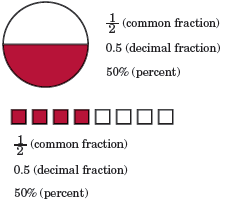# Fractional number

A fractional number is used to express a part of a whole or a group. A fractional number may also be used to express a ratio. A fractional number may be written in three different ways: As a common fraction, a decimal fraction, or a percent. Refer to the image for the examples below.

Example½, 0.5, or 50% of this circle is shaded.

½, 0.5, or 50% of these squares are shaded.

½, 0.5, or 50% are different names for the same fractional number